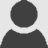# New PHPExcel release: 1.3.0

Edit on GitHub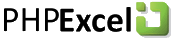The new version of PHPExcel has just been released, bringing 1.3.0 to the public. New features include formula calculation, inserting and removing columns/rows, auto-sizing columns, freezing panes, ...

One of the new features in PHPExcel is formula calculation. Just like Excel or any other spreadsheet application, PHPExcel now provides support for calculating certain cell values, using a formula. For example, the formula "=SUM(A1:A10)" evaluates to the sum of values in A1, A2, ..., A10.

Have a look at this: if you write the following line of code in the invoice demo included with PHPExcel, it evaluates to the value "64":

`\$objPHPExcel->getActiveSheet()->getCell('E11')->getCalculatedValue();`
`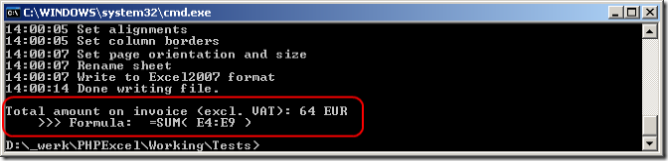`

Another nice feature of PHPExcel's formula parser, is that it can automatically adjust a formula when inserting/removing rows/columns. Here's an example: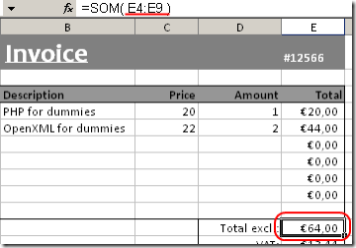You see that the formula contained in cell E11 is "SUM(E4:E9)". Now, when I write the following line of code, two new product lines are added:

`\$objPHPExcel->getActiveSheet()->insertNewRowBefore(7, 2);`
`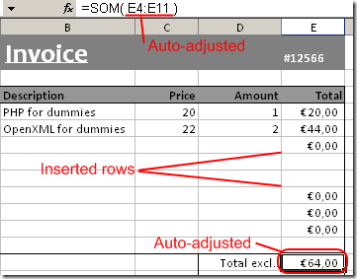`

Did you notice? The formula in the former cell E11 (now E13, as I inserted 2 new rows), changed to "SUM(E4:E11)". Also, the inserted cells duplicate style information of the previous cell, just like Excel's behaviour.

Curious about all this? Want to play with it? Find the source code download and demo code on www.phpexcel.net!

This is an imported post. It was imported from my old blog using an automated tool and may contain formatting errors and/or broken images.

Tags:

Updated: